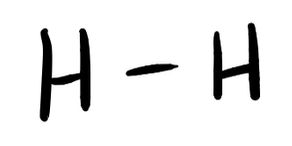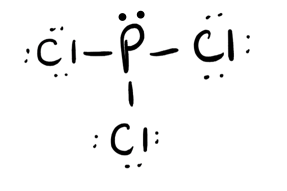# 3.8: Unit 3 Practice Problems

$$\newcommand{\vecs}{\overset { \rightharpoonup} {\mathbf{#1}} }$$ $$\newcommand{\vecd}{\overset{-\!-\!\rightharpoonup}{\vphantom{a}\smash {#1}}}$$$$\newcommand{\id}{\mathrm{id}}$$ $$\newcommand{\Span}{\mathrm{span}}$$ $$\newcommand{\kernel}{\mathrm{null}\,}$$ $$\newcommand{\range}{\mathrm{range}\,}$$ $$\newcommand{\RealPart}{\mathrm{Re}}$$ $$\newcommand{\ImaginaryPart}{\mathrm{Im}}$$ $$\newcommand{\Argument}{\mathrm{Arg}}$$ $$\newcommand{\norm}{\| #1 \|}$$ $$\newcommand{\inner}{\langle #1, #2 \rangle}$$ $$\newcommand{\Span}{\mathrm{span}}$$ $$\newcommand{\id}{\mathrm{id}}$$ $$\newcommand{\Span}{\mathrm{span}}$$ $$\newcommand{\kernel}{\mathrm{null}\,}$$ $$\newcommand{\range}{\mathrm{range}\,}$$ $$\newcommand{\RealPart}{\mathrm{Re}}$$ $$\newcommand{\ImaginaryPart}{\mathrm{Im}}$$ $$\newcommand{\Argument}{\mathrm{Arg}}$$ $$\newcommand{\norm}{\| #1 \|}$$ $$\newcommand{\inner}{\langle #1, #2 \rangle}$$ $$\newcommand{\Span}{\mathrm{span}}$$

### Question 1

The loss of electrons from an atom is called __________.

oxidation

The gain of electrons by an atom is called ___________.

Reduction

An atom with a charge (either positive or negative) is called __________.

ion

A positively charged atom is called _________.

cation

A negatively charged atom is called _________.

anion

### Question 2

Name the following ionic compounds.

MgS

NaCl

Ca3N2

KOH

AlPO4

magnesium sulfide

sodium chloride

calcium nitride

potassium hydroxide

aluminum phosphate

### Question 3

Given the following electronegativity values, determine the type of bond (polar covalent, nonpolar covalent, or ionic) which will occur between the atoms below.Li - F

C - O

C - H

Li - F: ionic bond

C - O: polar covalent bond

C - H: nonpoloar covalent

### Question 4

Given the name, write the formula for the following compounds.

calcium bromide

magnesium nitrate

iron (III) oxide

silver (I) sulfide

ammonium hydrogen phosphate

CaBr2

Mg(NO3)2

Fe2O3

Ag2S

(NH4)2HPO4

### Question 5

Write Lewis structures for the following:

(a)   H2

(b)   HBr

(c)   PCl3

(d)   SF2

(e)   C2H4

(f)   NO-

(g)  CN